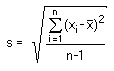The SDL Component Suite is an industry leading collection of components supporting scientific and engineering computing. Please visit the SDL Web site for more information....## CalcStatistics

 Unit: SDL_math2 Class: Declaration: procedure CalcStatistics (var NumData: longint; var MeanX, MeanY, StdevX, StdevY, MeanDiff, StdevDiff, rxy: Double);

The procedure CalcStatistics calculates the means, the standard deviations and the correlation coefficient of pairs of numbers. In addition, the mean and the standard deviation of the difference of the pairs are calculated using the following equation:In order to make use of CalcStatistics the user has first to initialize the internal registers (Init). Thereafter the pairs of x-y-values have to be entered to the register by using the procedure EnterStatValue. The procedure CalcStatistics evaluates the parameters using the data entered to far. It calculates the means and the standard deviations of x, y, and the difference x-y, as well as the correlation coefficient between x and y. In addition, the number of data points entered is returned in the parameter NumData.

This procedure can also be used to calculate the mean and standard deviation for a single series of data. In this case one of the values of the data pair [x,y] should be set to zero when using the procedure EnterStatValue. The calculated correlation coefficient is then, of course, invalid.

 Hint 1: Since it is only meaningful to calculate the standard deviation and the correlation coefficient with at least 3 data points the procedure CalcStatistics refuses to calculate these measures if less than 3 data points have been entered. The standard deviations and the correlation coefficient are set to 0.0 in this case.

 Hint 2: All the parameters returned by CalcStatistics can also be accessed individually by the appropriate properties (CorrCoeff, MeanDiff, MeanX, MeanY, NumData, StdDevDiff, StdDevX, StdDevY).

 Example: This property is used in the following example program (see http://www.lohninger.com/examples.html for downloading the code): multilinreg

Last Update: 2016-Aug-02